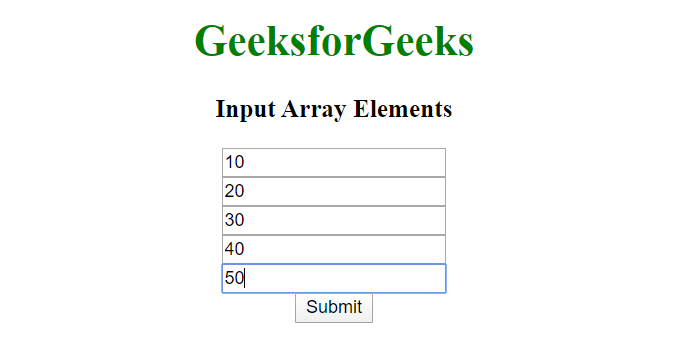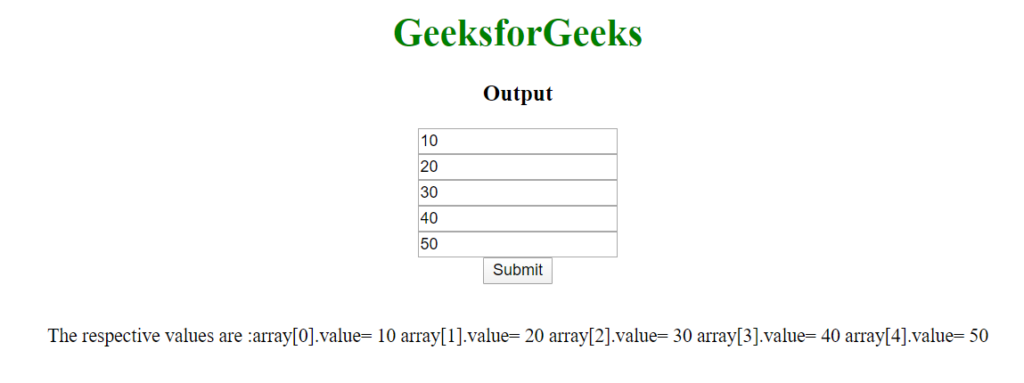Open in App
Not now

# How to get values from html input array using JavaScript ?

• Difficulty Level : Expert
• Last Updated : 10 May, 2022

This problem can be solved by using input tags having the same “name” attribute value that can group multiple values stored under one name which could later be accessed by using that name. To access all the values entered in input fields use the following method:

`var input = document.getElementsByName('array[]');`

The document.getElementsByName() method is used to return all the values stored under a particular name and thus making input variable an array indexed from 0 to number of inputs. The method document.getElementById().innerHTML is used to change the inner HTML of selected Id. So in this way, we can access the input values and convert them into the array and access it as you like. We have used form tag because it is generally a good practice to group all the input elements together inside a form otherwise in the present scenario it is completely optional.

Example:

## html

 `` `<``html` `lang="en" dir="ltr">`   `<``head``>` `    ``<``meta` `charset="utf-8">` `    ``<``title``>` `        ``How to get values from html input` `        ``array using JavaScript ?` `    ``` ``   `<``body` `style="text-align: center;">` `    `  `    ``<``h1` `style="color: green;">` `        ``GeeksforGeeks` `    ```   `    ``<``h3` `id="po">Input Array Elements` `    ``<``form` `class="" action="index.html" method="post">` `        ``<``input` `type="text" name="array[]" value="" /><``br``>` `        ``<``input` `type="text" name="array[]" value="" /><``br``>` `        ``<``input` `type="text" name="array[]" value="" /><``br``>` `        ``<``input` `type="text" name="array[]" value="" /><``br``>` `        ``<``input` `type="text" name="array[]" value="" /><``br``>` `        ``<``button` `type="button" name="button" onclick="Geeks()">` `            ``Submit` `        ``` `    ``` `    ``<``br``>`   `    ``<``p` `id="par">`   `    ``<``script` `type="text/javascript">` `        ``var k = "The respective values are :";` `        ``function Geeks() {` `            ``var input = document.getElementsByName('array[]');`   `            ``for (var i = 0; i < ``input.length``; i++) {` `                ``var ``a` `= ``input``[i];` `                ``k` `= k + "array[" + i + "].value= "` `                                   ``+ a.value + " ";` `            ``}`   `            ``document.getElementById("par")``.innerHTML` `= ``k``;` `            ``document.getElementById("po").innerHTML = "Output";` `        ``}` `    ``` ``   ``

Output:

• Input Array Elements:• Output Array Elements:JavaScript is best known for web page development but it is also used in a variety of non-browser environments. You can learn JavaScript from the ground up by following this JavaScript Tutorial and JavaScript Examples.

My Personal Notes arrow_drop_up
Related Articles